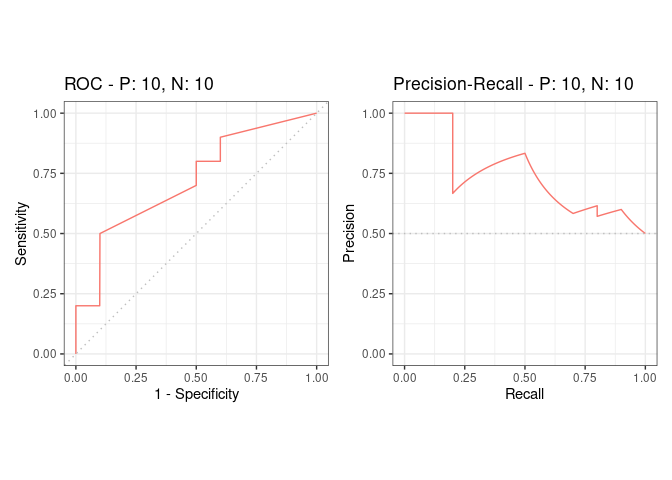The aim of the precrec package is to provide an integrated platform that enables robust performance evaluations of binary classifiers. Specifically, precrec offers accurate calculations of ROC (Receiver Operator Characteristics) and precision-recall curves. All the main calculations of precrec are implemented with C++/Rcpp.

## Documentation

• Package website – GitHub pages that contain all precrec documentation.

• Introduction to precrec – a package vignette that contains the descriptions of the functions with several useful examples. View the vignette with vignette("introduction", package = "precrec") in R. The HTML version is also available on the GitHub Pages.

• Help pages – all the functions including the S3 generics except for print have their own help pages with plenty of examples. View the main help page with help(package = "precrec") in R. The HTML version is also available on the GitHub Pages.

## Six key features of precrec

### 1. Accurate curve calculations

precrec provides accurate precision-recall curves.

• Non-linear interpolation
• Elongation to the y-axis to estimate the first point when necessary
• Use of score-wise threshold values instead of fixed bins

precrec also calculates AUC scores with high accuracy.

### 2. Super fast

precrec calculates curves in a matter of seconds even for a fairly large dataset. It is much faster than most other tools that calculate ROC and precision-recall curves.

### 3. Various evaluation metrics

In addition to precision-recall and ROC curves, precrec offers basic evaluation measures.

• Error rate
• Accuracy
• Specificity
• Sensitivity, true positive rate (TPR), recall
• Precision, positive predictive value (PPV)
• Matthews correlation coefficient
• F-score

### 4. Confidence interval band

precrec calculates confidence intervals when multiple test sets are given. It automatically shows confidence bands about the averaged curve in the corresponding plot.

### 5. Calculation of partial AUCs and visualization of partial curves

precrec calculates partial AUCs for specified x and y ranges. It can also draw partial ROC and precision-recall curves for the specified ranges.

### 6. Supporting functions

precrec provides several useful functions that lack in most other evaluation tools.

• Handling multiple models and multiple test sets
• Handling tied scores and missing scores
• Pre- and post-process functions of simple data preparation and curve analysis

## Installation

• Install the release version of precrec from CRAN with install.packages("precrec").

• Alternatively, you can install a development version of precrec from our GitHub repository. To install it:

1. Make sure you have a working development environment.

• Windows: Install Rtools (available on the CRAN website).
• Mac: Install Xcode from the Mac App Store.
• Linux: Install a compiler and various development libraries (details vary across different flavors of Linux).
2. Install devtools from CRAN with install.packages("devtools").

3. Install precrec from the GitHub repository with devtools::install_github("evalclass/precrec").

## Functions

The precrec package provides the following six functions.

Function Description
evalmod Main function to calculate evaluation measures
mmdata Reformat input data for performance evaluation calculation
join_scores Join scores of multiple models into a list
join_labels Join observed labels of multiple test datasets into a list
create_sim_samples Create random samples for simulations
format_nfold Create n-fold cross validation dataset from data frame

Moreover, the precrec package provides nine S3 generics for the S3 object created by the evalmod function. N.B. The R language specifies S3 objects and S3 generic functions as part of the most basic object-oriented system in R.

S3 generic Package Description
print base Print the calculation results and the summary of the test data
as.data.frame base Convert a precrec object to a data frame
plot graphics Plot performance evaluation measures
autoplot ggplot2 Plot performance evaluation measures with ggplot2
fortify ggplot2 Prepare a data frame for ggplot2
auc precrec Make a data frame with AUC scores
part precrec Calculate partial curves and partial AUC scores
pauc precrec Make a data frame with pAUC scores
auc_ci precrec Calculate confidence intervals of AUC scores

## Examples

Following two examples show the basic usage of precrec functions.

### ROC and Precision-Recall calculations

The evalmod function calculates ROC and Precision-Recall curves and returns an S3 object.

library(precrec)

# Load a test dataset
data(P10N10)

# Calculate ROC and Precision-Recall curves
sscurves <- evalmod(scores = P10N10$scores, labels = P10N10$labels)

### Visualization of the curves

The autoplot function outputs ROC and Precision-Recall curves by using the ggplot2 package.

# The ggplot2 package is required
library(ggplot2)

# Show ROC and Precision-Recall plots
autoplot(sscurves)## Citation

Precrec: fast and accurate precision-recall and ROC curve calculations in R

Takaya Saito; Marc Rehmsmeier

Bioinformatics 2017; 33 (1): 145-147.